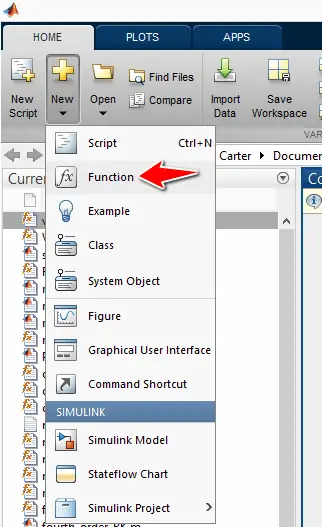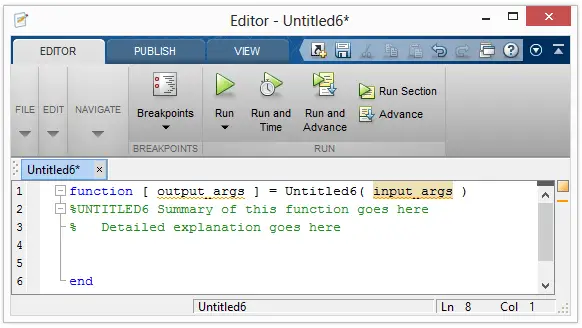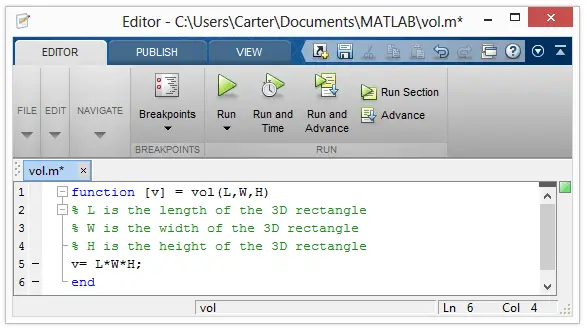Home MathLab Write a Function That Find the Volume of a 3D Rectangle in Matlab

# Write a Function That Find the Volume of a 3D Rectangle in Matlab

There is a function behind every Matlab code that performs a computational task, and here is how you can create your own function that will help you go faster while working with Matlab.

We will be creating a simple one-line code function in this post, just to help you have a glimpse into how creating functions in Matlab

## Write a function in Matlab

Here is what you do when writing your own function in Matlab.

Open a new function fileHere is what appear on the next windowIn Matlab, the section after the % is considered as a comment (the section that appears in green in the window above)

Here is how we need to structure our Matlab function

[php]function [v] = vol(L,W,H)
% L is the length of the 3D rectangle
% W is the width of the 3D rectangle
% H is the height of the 3D rectangle
v= L*W*H;
end[/php]

You need to copy the whole code above and paste it in the file, Save the file with the name vol.m and make sure not to change the directory Matlab will guide you to.

Here is how it will look## How to use the Matlab function we have just created

Every time you will need to compute the such a volume, simply type the following code

`vol(L,W,H)`

where L, W, And H will be real numbers.

#### Example

Let’s say, we need the volume of a 3D rectangle with L, W and H being respectively 10, 3, 15, we can simply type:

`vol(10,3,15)`

And Matlab will call the function vol, compute the volume of the 3D rectangle and display the following.If you are working in an exercise where you will often need this volume, you can just use this function instead of actually computing manually the volume each time you need it. It might not look very important in this exercise, but when you have a complex operation you need to perform often in Matlab, creating a function doing it automatically will be time-efficient and necessary.

Using this technique, you can create more complex functions in Matlab and have an easier life while using them. Learn more about functions in Matlab.